Courses

Test: Name Reaction Level - 2

30 Questions MCQ Test Organic Chemistry | Test: Name Reaction Level - 2

Description
Attempt Test: Name Reaction Level - 2 | 30 questions in 60 minutes | Mock test for Chemistry preparation | Free important questions MCQ to study Organic Chemistry for Chemistry Exam | Download free PDF with solutions
QUESTION: 1

Solution:
QUESTION: 2

The major product formed in the following reaction is: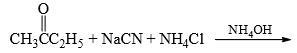Solution:

Nucleophilic addition of cyanide gives product B and then by NH4OH substitution of OH by NH2 gives product C.

QUESTION: 3

The compound having the formula,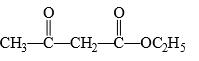Solution:
QUESTION: 4

Fehling’s solution can be used for distinguishing between:

Solution:
QUESTION: 5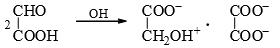​The above reaction can said to be an example of:

Solution:
QUESTION: 6

Ninhydrin has three keto groups, which of the keto group is expected to be hydrated most easily: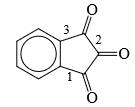Solution:
QUESTION: 7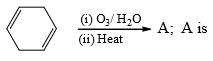Solution:
QUESTION: 8

Which of the following does not react with sodium bisulphate: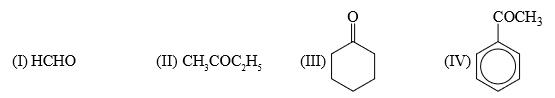Solution:
QUESTION: 9

Which of the following reagent can be used for carrying out the reaction outlined below: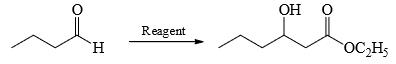Solution:
QUESTION: 10

Which of the following statement is false:

Solution:
QUESTION: 11

Which of the following compounds is not formed in iodoform reaction of acetone?

Solution:
QUESTION: 12

Which of the following is not formed in Iodoform reaction:

Solution:
QUESTION: 13

The major product formed in the following reaction is: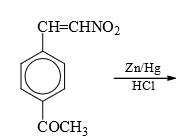Solution:
QUESTION: 14

The major product formed in the following reaction is: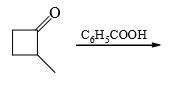Solution:
QUESTION: 15

Which of the following statement is true:

Solution:
QUESTION: 16

What is the main product whenSolution:
QUESTION: 17

Predict the nature of end product in the following reaction: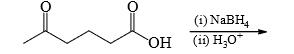Solution:
QUESTION: 18

Salicylic acid is treated with bromine under two different conditions: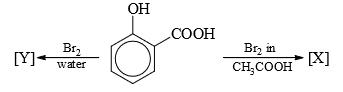Predict the nature of [X] and [Y] in the following reactions

Solution:
QUESTION: 19

A carboxylic acid can best be converted into acid chloride by using:

Solution:

Use of SOCl2 and CICOCOCI forms gaseous by products which can be easily removed, giving better yield of RCOCI. Further, oxalyl chIoride is particularly easy to use becasue any excess of it can be easily evaporated due to its low b.p. (62C)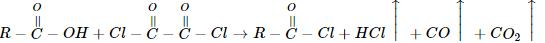QUESTION: 20

Which of the following does not reduce C6H5NO2 to aniline:

Solution:
QUESTION: 21

What could be the reagent for the following reaction:​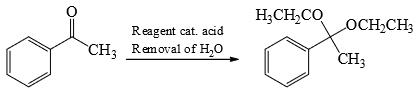Solution:
QUESTION: 22

What could be the product for the following reaction: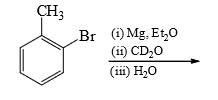Solution:
QUESTION: 23

Which ester will not give a good yield of the Claisen condensation product with NaOEt in EtOH?

Solution:

The Claisen condensation is reversible and it is formation of a stabilized enolate of the product which leads to a high yield at equilibrium. When the product cannot give a stabilized enolate, the yield will be poor.

QUESTION: 24

What could be the product for the following reaction: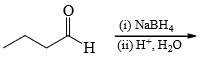Solution:
QUESTION: 25

What could be the product for the following reaction: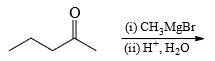Solution:
QUESTION: 26

What could be the product for the following reaction: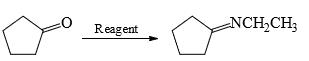Solution:
QUESTION: 27

The major product formed in the following reaction is: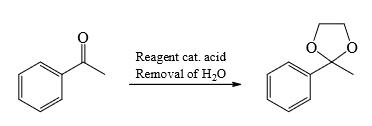Solution:

HOCH2CH2OH, ethylene glycol will react with acid to give 5 membered ring with the carbonyl group.

QUESTION: 28

The major product formed in the following reaction is: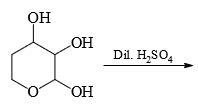Solution:
QUESTION: 29

The major product formed in the following reaction is: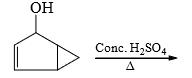Solution:
QUESTION: 30

The major product formed in the following reaction is: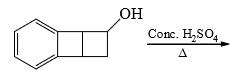Solution:Use Code STAYHOME200 and get INR 200 additional OFF Use Coupon Code

Track your progress, build streaks, highlight & save important lessons and more!

Similar ContentRelated tests